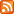lists.arthurdejong.org# Re: nslcd eats up all memory

• From: Peter Slickers <pesli [at] web.de>
• To: nss-pam-ldapd-users [at] lists.arthurdejong.org
• Subject: Re: nslcd eats up all memory
• Date: Mon, 08 Nov 2010 23:35:47 +0100

```On 03.11.2010 23:02, Arthur de Jong wrote:
```
```
Do you also use the PAM module?

```
With active directory the preferred protocol for authentication is Kerberos. Thus I do not use the PAM module provided by the nslcd package but rather the krb5 PAM module.
```
```
```LDAP backend
Active Directory with IDMU installed (Windows 2008 server)
```
```
Can you give an indication of the number of users and groups in there?

```
```about 300 user and 40 group objects

```
```
Can you try running nslcd under valgrind:
valgrind --leak-check=full /usr/sbin/nslcd -d
```
```
```
I have started nslcd with valgrind on a test server with low traffic. After running it for a few minutes I get the following:
```
==11871==
==11871== HEAP SUMMARY:
==11871==     in use at exit: 425,442 bytes in 371 blocks
==11871==   total heap usage: 4,810 allocs, 4,439 frees, 1,623,213 bytes
allocated
==11871==
==11871== 35 bytes in 5 blocks are definitely lost in loss record 12 of 42
==11871==    at 0x4023F50: malloc (vg_replace_malloc.c:236)
==11871==    by 0x804C073: log_newsession (log.c:139)
==11871==    by 0x804B4EF: worker (nslcd.c:364)
==11871==    by 0x4197E7D: clone (clone.S:130)
==11871==
==11871== 46 bytes in 1 blocks are definitely lost in loss record 13 of 42
==11871==    at 0x4024046: realloc (vg_replace_malloc.c:525)
==11871==    by 0x42197AF: ber_memrealloc_x (in /usr/lib/liblber-2.4.so.2.5.6)
==11871==    by 0x409E5C8: ldap_domain2hostlist (in
/usr/lib/libldap_r-2.4.so.2.5.6)
==11871==    by 0x8051EAA: cfg_init (cfg.c:1160)
==11871==    by 0x804AB2F: main (nslcd.c:631)
==11871==
==11871== 100,416 (480 direct, 99,936 indirect) bytes in 6 blocks are
definitely lost in loss record 38 of 42
==11871==    at 0x402328F: calloc (vg_replace_malloc.c:467)
==11871==    by 0x4218CDF: ber_memcalloc_x (in /usr/lib/liblber-2.4.so.2.5.6)
==11871==    by 0x408F0A0: ldap_send_server_request (in
/usr/lib/libldap_r-2.4.so.2.5.6)
==11871==    by 0x408FEB1: ldap_chase_v3referrals (in
/usr/lib/libldap_r-2.4.so.2.5.6)
==11871==    by 0x407B0A3: ldap_result (in /usr/lib/libldap_r-2.4.so.2.5.6)
==11871==    by 0x804E6FE: myldap_get_entry (myldap.c:1059)
==11871==    by 0x8057266: nslcd_passwd_byuid (passwd.c:416)
==11871==    by 0x804BBC8: worker (nslcd.c:411)
==11871==    by 0x4197E7D: clone (clone.S:130)
==11871==
==11871== 117,152 (560 direct, 116,592 indirect) bytes in 7 blocks are
definitely lost in loss record 40 of 42
==11871==    at 0x402328F: calloc (vg_replace_malloc.c:467)
==11871==    by 0x4218CDF: ber_memcalloc_x (in /usr/lib/liblber-2.4.so.2.5.6)
==11871==    by 0x408F0A0: ldap_send_server_request (in
/usr/lib/libldap_r-2.4.so.2.5.6)
==11871==    by 0x408FEB1: ldap_chase_v3referrals (in
/usr/lib/libldap_r-2.4.so.2.5.6)
==11871==    by 0x407B0A3: ldap_result (in /usr/lib/libldap_r-2.4.so.2.5.6)
==11871==    by 0x804E6FE: myldap_get_entry (myldap.c:1059)
==11871==    by 0x8054AFE: nslcd_group_byname (group.c:281)
==11871==    by 0x804BC0D: worker (nslcd.c:399)
==11871==    by 0x4197E7D: clone (clone.S:130)
==11871==
==11871== 200,832 (960 direct, 199,872 indirect) bytes in 12 blocks are
definitely lost in loss record 42 of 42
==11871==    at 0x402328F: calloc (vg_replace_malloc.c:467)
==11871==    by 0x4218CDF: ber_memcalloc_x (in /usr/lib/liblber-2.4.so.2.5.6)
==11871==    by 0x408F0A0: ldap_send_server_request (in
/usr/lib/libldap_r-2.4.so.2.5.6)
==11871==    by 0x408FEB1: ldap_chase_v3referrals (in
/usr/lib/libldap_r-2.4.so.2.5.6)
==11871==    by 0x407B0A3: ldap_result (in /usr/lib/libldap_r-2.4.so.2.5.6)
==11871==    by 0x804E6FE: myldap_get_entry (myldap.c:1059)
==11871==    by 0x8057A9E: nslcd_passwd_byname (passwd.c:401)
==11871==    by 0x804BB8C: worker (nslcd.c:410)
==11871==    by 0x4197E7D: clone (clone.S:130)
==11871==
==11871== LEAK SUMMARY:
==11871==    definitely lost: 2,081 bytes in 31 blocks
==11871==    indirectly lost: 416,400 bytes in 275 blocks
==11871==      possibly lost: 0 bytes in 0 blocks
==11871==    still reachable: 6,961 bytes in 65 blocks
==11871==         suppressed: 0 bytes in 0 blocks
==11871== Reachable blocks (those to which a pointer was found) are not shown.
==11871== To see them, rerun with: --leak-check=full --show-reachable=yes
==11871==
==11871== For counts of detected and suppressed errors, rerun with: -v
==11871== ERROR SUMMARY: 5 errors from 5 contexts (suppressed: 54 from 9)

```
```Typical messages in /var/log/daemon.log:
Nov  2 13:46:16 conan nslcd: [87739c]
Nov  2 13:47:05 conan nslcd: [294e43]
nslcd_group_byname(Domänen-Benutzer): invalid group name
```
```
```
```The other ones are due to the umlaut in the name. POSIX has pretty
strict limits as to what characters should be part of group names.
```
```
```
The two accounts 'Domänen-Admins' and 'Domänen-Benutzer' are pure Windows accounts which do not have any Posix attributes. Samba clients are frequently asking for them because 'Domänen-Benutzer' is the primary Windows group of most users. If nslcd would try to lookup their Posix attributes via LDAP this would result an error.
```--
Peter Slickers

--
To unsubscribe send an email to
nss-pam-ldapd-users-unsubscribe@lists.arthurdejong.org or see
http://lists.arthurdejong.org/nss-pam-ldapd-users```